# Heat Exchangers (Part - 6) Chemical Engineering Notes | EduRev

## Chemical Engineering : Heat Exchangers (Part - 6) Chemical Engineering Notes | EduRev

The document Heat Exchangers (Part - 6) Chemical Engineering Notes | EduRev is a part of the Chemical Engineering Course Heat Transfer for Engg..
All you need of Chemical Engineering at this link: Chemical Engineering

8.2.9 Calculation and designing of the heat exchanger
8.2.9.1 Double-pipe heat exchanger

The following steps may be used to design a double-pipe heat exchanger

1. Calculate LMTD from the known terminal temperatures.
2. Diameter of the inner and outer pipes may be selected from the standard pipes from the literature (generally available with the vendor and given in the books). The selection thumb rule is the consideration of higher fluid velocity and low pressure drop in the pipe.
3. Calculate the Reynolds number and evaluate the heat transfer coefficient, hi, using the co-relations given in the chapter.
4. Similarly, calculate the Reynolds number of the fluid flowing through annulus. Calculation the equivalent diameter of the annulus and find the outside heat transfer coefficient, ho.
5. Using hi and ho, calculate the overall heat transfer coefficients. Note that it will be a clean overall heat transfer coefficient. In order to find design outside heat transfer coefficient using a suitable dirt factor or fouling factor. The tube fouling factor is suggested by TEMA (table 8.1).

The calculations are based on trial and error. If the heat transfer coefficient comes out to be very small or the pressure drop comes out to be very high, this procedure to be redone for different set of diameters in the step1.

8.2.9.2 Shell and tube heat exchanger
The shell and tube heat exchanger also involves trial and error but it is not as simple as in case of double pipe heat exchanger.

The design of shell and tube heat exchanger includes,
a: heat transfer required for the given heat duty
b: tube diameter, length, and number,
c: shell diameter,
d: no of shell and tube passes,
e: tube arrangement on the tube sheet and its layout, and
f: baffle size, number and spacing of the baffles.

The calculation of LMTD can be done if the terminal temperatures are known. However, the design heat transfer co-efficient  (i.e., heat transfer co-efficient including fouling factor) and the area are dependent on each other and thus challenges involve for the estimation. The also depends upon Reynolds number, which depends upon the liquid flow rate, sizes and the number of tubes. Therefore, is a function of diameter and the no of tubes and the parameter provides the area.

Moreover, can also be calculated is based on shell side co-efficient but then it requires tube number, diameters and pitch. Thus, the above discussion shows that and A are not fully explicit and requires trial and error method of calculation.
The guideline for shell-and-tube calculation is shown in below,

1. energy balance and exchanger heat duty calculation,
2. find all the thermo-physical properties of the fluid,
3. take initial guess for shell-and-tube passes,
4. calculate LMTD and FT,
5. assume (or select) Udirty, based on the outside tube area. Calculate corresponding heat transfer area, A.
6. Select tube diameter, wall thickness and the tube length. Based on this values and heat transfer area, find out the no of the tubes required.
7. Assume the tube pitch and assume diameter of the shell, which can accumulate the no of tube. Now, select the tube-sheet layout.
8. Select the baffle design.
9. Estimate hi and ho, if the estimated shell-side heat transfer coefficient (ho) appears to be small; the baffles at a close distance may be tried. If the tube side co-efficient (hi) is low, the number of tube passes to be reconsidered such that the Reynolds number increases (for a reasonable Δp) and henceforth hi.
10. Evaluate Uclean on the outside tube area basis. Select a suitable fouling factor (Rd) and find Udirty
11. Compare Udirty and A values with the values assumed in step (5). If Acalculate ≥ Aassumed, it may be acceptable. Otherwise a new configuration in terms of the size and no of the tubes and tube passes, shell diameter is assumed and recalculation be done
12. Calculate the tube-side and shell side Δp. If Δp is more than the allowable limit, the re-calculate after suitable adjustment has to be done.

Illustration
A heat transfer fluid is leaving a reactor at a rate of 167 kg/s at 85°C. The fluid is to be cooled to 50°C before it can be recycled to the reactor. Water is available at 30°C to cool the fluid in a 1-2 pass heat exchanger having heat transfer area of 15 m2. The water, which is being used to cool the fluid, must not be heated to above 38°C at the exit of the heat exchanger. The overall heat transfer co-efficient of 400 Kcal/hm2°C can be used for the heat exchanger. The water flows through the shell and the oil flows through the tubes. The specific heat of the fluid may be taken as 0.454 kcal/kg°C. Find out whether the heat exchanger would be suitable for the given heat duty?

Solution:
It is given,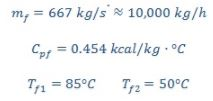f : hot stream (fluid)

c : cold stream (water)

Energy balance across the heat exchanger will be,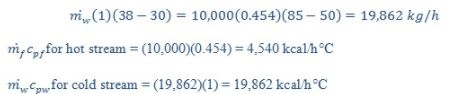Thus the minimum stream will be the hot stream.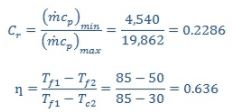Putting the values in the eq. 8.19,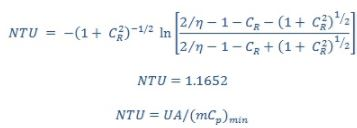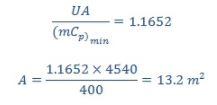The area 13.2 m2 found is less than the available area (15 m2). Therefore, the given heat exchanger will perform the required heat duty.

Offer running on EduRev: Apply code STAYHOME200 to get INR 200 off on our premium plan EduRev Infinity!

## Heat Transfer for Engg.

46 videos|60 docs|59 tests

,

,

,

,

,

,

,

,

,

,

,

,

,

,

,

,

,

,

,

,

,

;